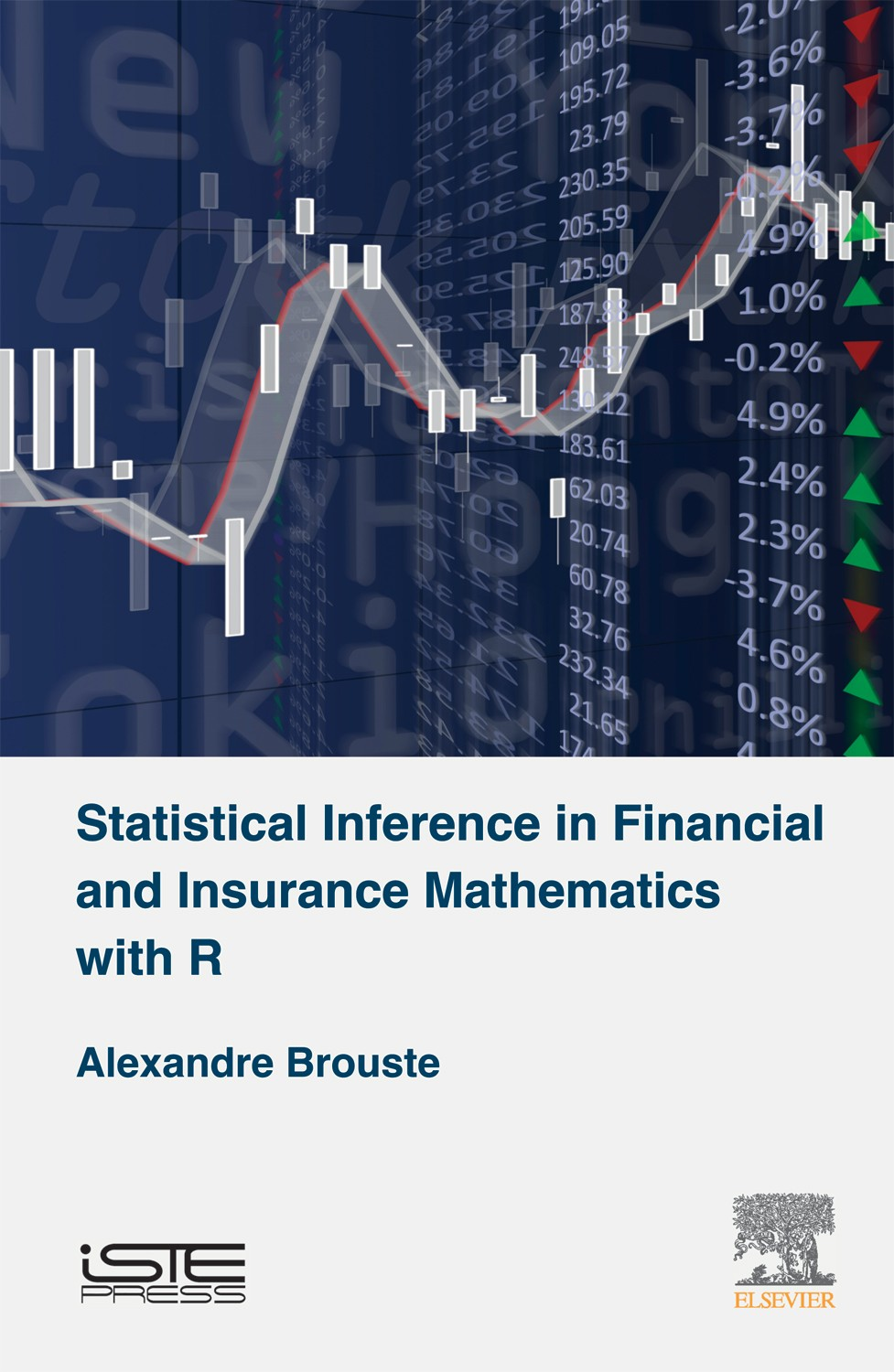pages
202
ISBN
9781785480836

Finance and insurance companies are facing a wide range of parametric statistical problems. Statistical experiments generated by a sample of independent and identically distributed random variables are frequent and well understood, especially those consisting of probability measures of an exponential type. However, the aforementioned applications also offer non-classical experiments implying observation samples of independent but […]

Available at your bookseller and Amazon

Finance and insurance companies are facing a wide range of parametric statistical problems. Statistical experiments generated by a sample of independent and identically distributed random variables are frequent and well understood, especially those consisting of probability measures of an exponential type. However, the aforementioned applications also offer non-classical experiments implying observation samples of independent but not identically distributed random variables or even dependent random variables.

Three examples of such experiments are treated in this book. First, the Generalized Linear Models are studied. They extend the standard regression model to non-Gaussian distributions. Statistical experiments with Markov chains are considered next. Finally, various statistical experiments generated by fractional Gaussian noise are also described.

In this book, asymptotic properties of several sequences of estimators are detailed. The notion of asymptotical efficiency is discussed for the different statistical experiments considered in order to give the proper sense of estimation risk. Eighty examples and computations with R software are given throughout the text.

Part 1. Inference in Parametric Statistical Experiments 1. Statistical Experiments. 2. Statistical Inference. 3. Asymptotic Efficiency. Part 2. Statistical Inference for Insurance 4. Statistical Experiments in Insurance. Part 3. Statistical Inference for Finance 5. Homogeneous Diffusion Processes. 6. Statistical Experiments in Finance.

## Alexandre Brouste

Alexandre Brouste is Professor in Statistics with the Institute of Risk and Insurance at Le Mans University, France.